## 简介概要

Key words：counter current extraction; theory of optimization; production cost; extraction factor; rare earth

Abstract: Based on the optimization goal searching for extraction parameters of minimun production cost, a novel equation for calculating optimized extraction factor of minimum production cost in fractional extraction was proposed. For short-cut calculation, new equations for determining the optimized extraction factors of minimum production cost in both the extraction and scrubbing sections were derived from above novel equation. These equations and theory have further developed the theory on equations of optimized extraction factors.

## 详情信息展示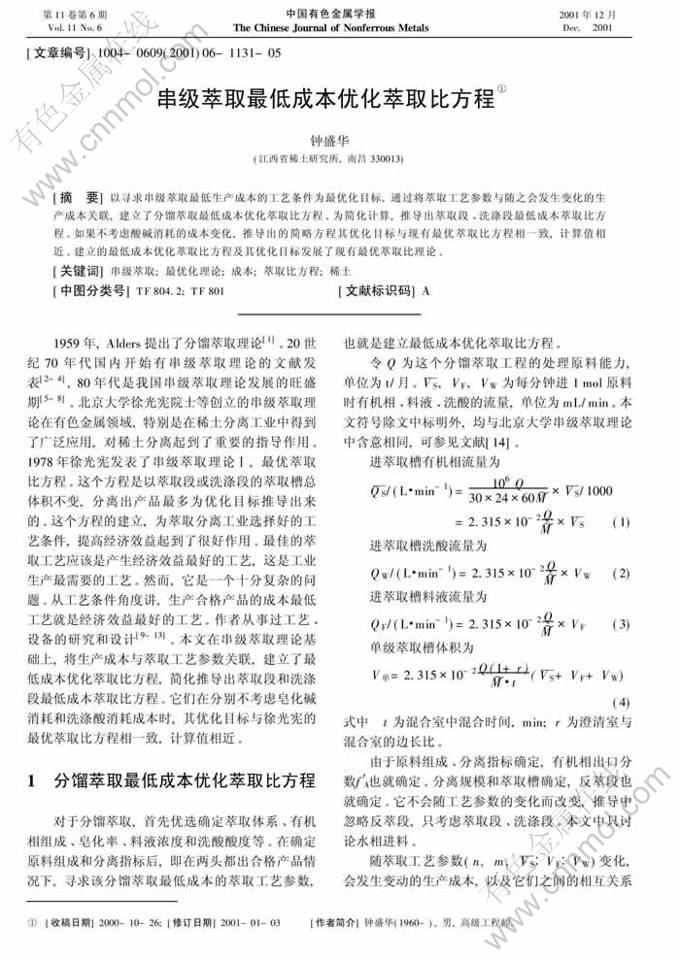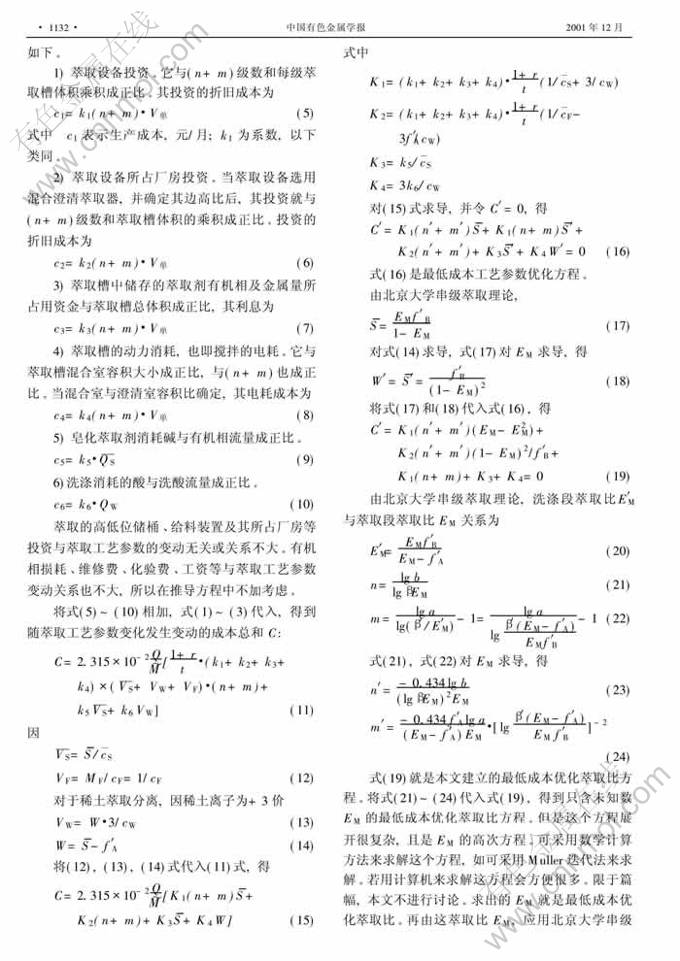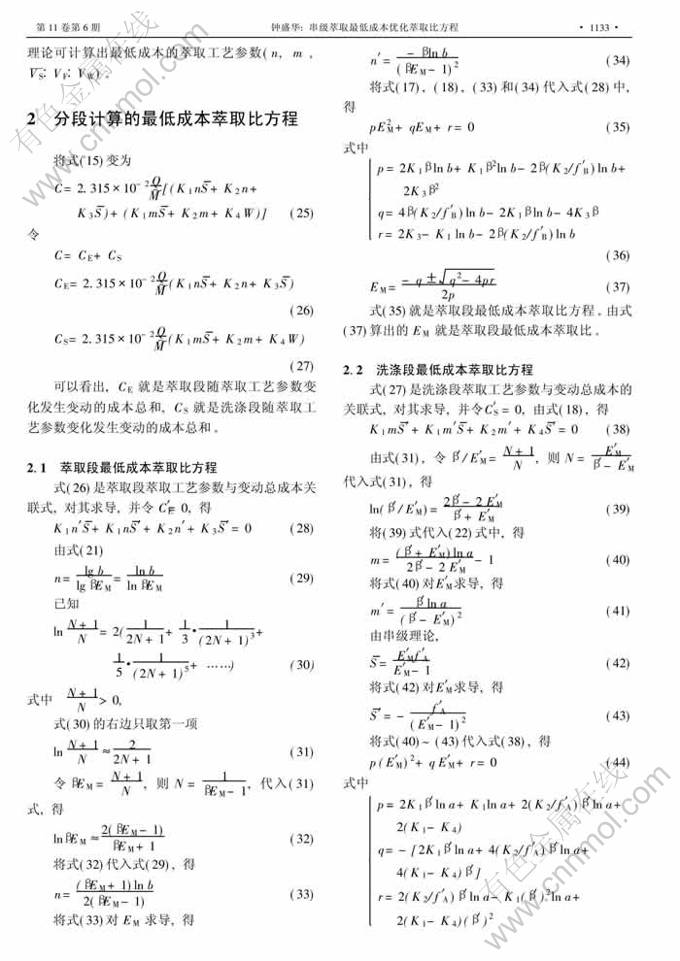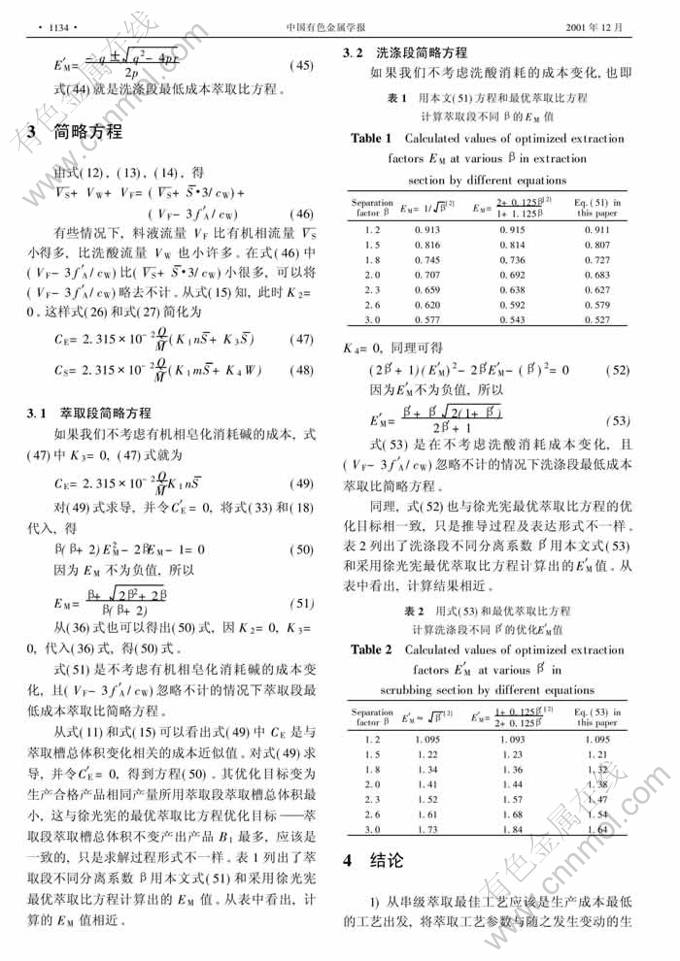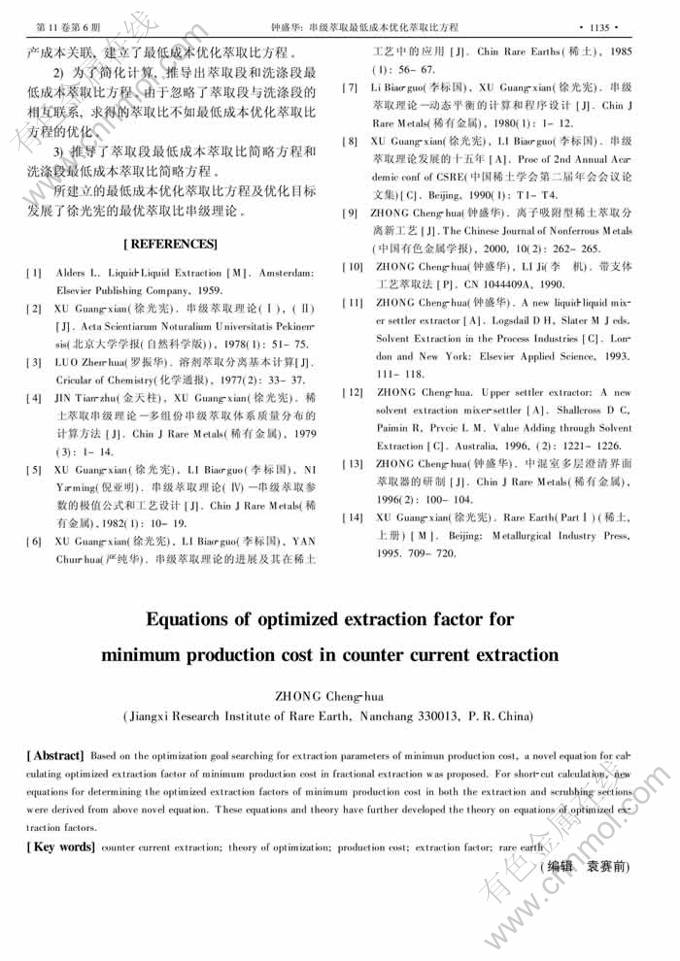<上一页 1 下一页 >